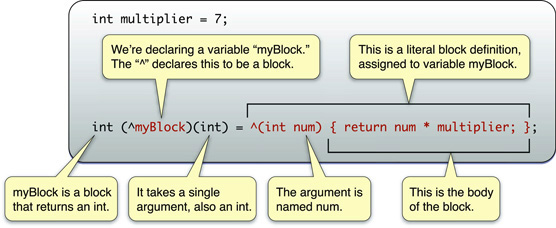## Blocks

Blocks是C语言的新拓展，一句话来概括的话，可以理解为“anonymous functions together with automatic (local) variables.” Blocks有点像函数，但是它可以在其它函数或方法中进行声明和定义，同时它还是匿名的（匿名函数），并可以捕获其所在作用域中的变量（闭包特性)。

``````int multiplier = 7;
int (^myBlock)(int) = ^(int num) {
return num * multiplier;
};
``````block有点像函数，事实上它确实可以像函数一样使用

``````int multiplier = 7;
int (^myBlock)(int) = ^(int num) {
return num * multiplier;
};

printf("%d", myBlock(3));
// prints "21"
``````

block也可以作为函数的参数

``````char *myCharacters = { "TomJohn", "George", "Charles Condomine" };

qsort_b(myCharacters, 3, sizeof(char *), ^(const void *l, const void *r) {
char *left = *(char **)l;
char *right = *(char **)r;
return strncmp(left, right, 1);
});
``````

block有闭包的特性，所以可以捕获所在作用域的变量

``````void testBlock() {
int a = 1;
int b = 2;
int (^aBlock)(void) = ^ { return a + b; };
printf("%d\n", aBlock());   // 输出 3
a = 0;
printf("%d\n", aBlock());   // 还是输出 3
}
``````

``````void testBlock() {
__block int a = 1;
int b = 2;
int (^aBlock)(void) = ^ { return a + b; };
a = 0;
printf("%d\n", aBlock());   // 输出 2
}
``````

Blocks 通常代表一个很小、自包的代码片段。因此它们作为封装的工作单元在并 发执行,或在一个集合项上,或当其他操作完成时的回调的时候非常实用。

• 如果你通过引用来访问一个实例变量,self 会被 retain。
• 如果你通过值来访问一个实例变量,那么变量会被 retain。